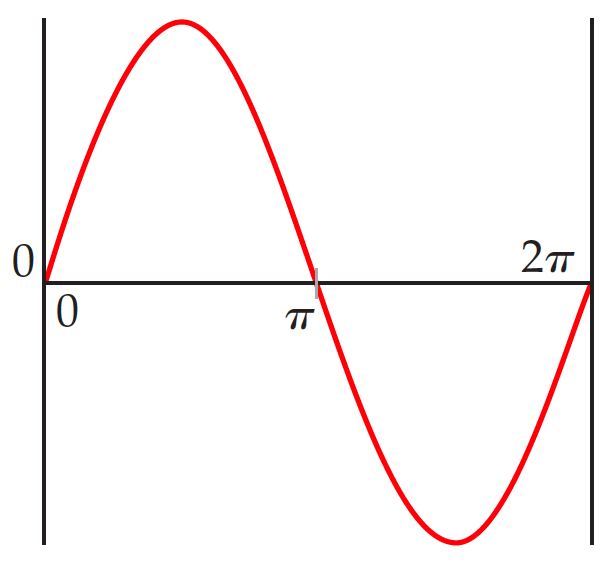# Problem: Consider a fictitious one-dimensional system with one electron. The wave function for the electron, drawn below, is Ψ(x) = sin x from x = 0 to x = 2π..Sketch the probability density, Ψ2(x), from x = 0 to x = 2π..

###### FREE Expert Solution
86% (339 ratings)
###### Problem Details

Consider a fictitious one-dimensional system with one electron. The wave function for the electron, drawn below, is Ψ(x) = sin x from x = 0 to x = 2π..Sketch the probability density, Ψ2(x), from x = 0 to x = 2π..